Warning: include(../header.php): Failed to open stream: No such file or directory in /var/www/html/retro/bbdep2010/ImagesMovies.php on line 6

Warning: include(): Failed opening '../header.php' for inclusion (include_path='.:') in /var/www/html/retro/bbdep2010/ImagesMovies.php on line 6

### Components of 2010 Rotamer Library:

 Rotamerprobability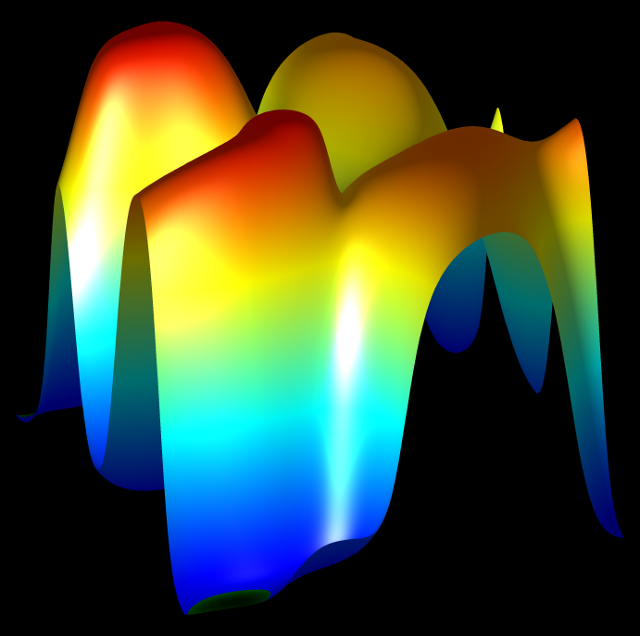χ mean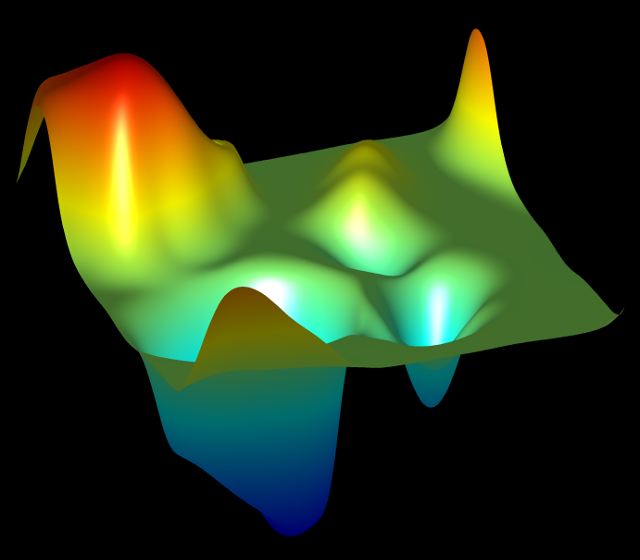χ standarddeviation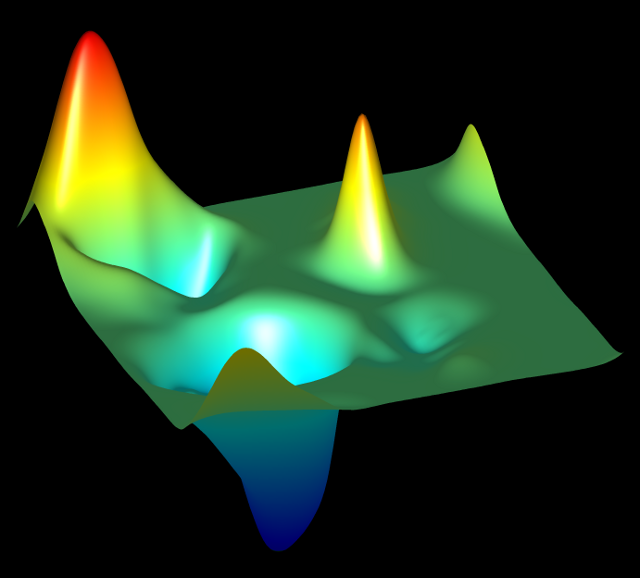Non-rotameric χn density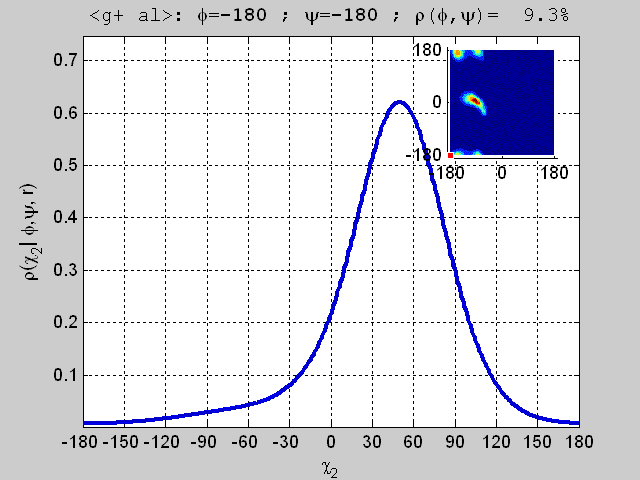### Images and Movies from 2010 Rotamer Libraryfor All Residue Types:

RES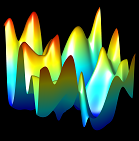5%-smoothed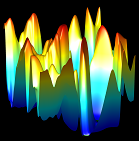0%-smoothed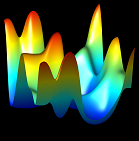20%-smoothed

ALL

ALL

Browse folders

Browse folders

Browse folders

ARG

ASN

 Rotamer probabilities χ1 mean χ1 standard deviation χ2 density, Mpg, Gif χ2 density, < t> Mpg, Gif χ2 density, Mpg, Gif
 Rotamer probabilities χ1 mean χ1 standard deviation χ2 density, χ2 density, < t> χ2 density,
 Rotamer probabilities χ1 mean χ1 standard deviation χ2 density, χ2 density, < t> χ2 density,

ASP

 Rotamer probabilities χ1 mean χ1 standard deviation χ2 density, Mpg, Gif χ2 density, < t> Mpg, Gif χ2 density, Mpg, Gif
 Rotamer probabilities χ1 mean χ1 standard deviation χ2 density, χ2 density, < t> χ2 density,
 Rotamer probabilities χ1 mean χ1 standard deviation χ2 density, χ2 density, < t> χ2 density,

CYS

GLN

 Rotamer probabilities χ1 mean χ1 standard deviation χ2 mean χ2 standard deviation χ3 density, Mpg, Gif χ3 density, Mpg, Gif χ3 density, Mpg, Gif χ3 density, < t,g+> Mpg, Gif χ3 density, < t,t > Mpg, Gif χ3 density, < t,g-> Mpg, Gif χ3 density, Mpg, Gif χ3 density, Mpg, Gif χ3 density, Mpg, Gif
 Rotamer probabilities χ1 mean χ1 standard deviation χ2 mean χ2 standard deviation χ3 density, χ3 density, χ3 density, χ3 density, < t,g+> χ3 density, < t,t > χ3 density, < t,g-> χ3 density, χ3 density, χ3 density,
 Rotamer probabilities χ1 mean χ1 standard deviation χ2 mean χ2 standard deviation χ3 density, χ3 density, χ3 density, χ3 density, < t,g+> χ3 density, < t,t > χ3 density, < t,g-> χ3 density, χ3 density, χ3 density,

GLU

 Rotamer probabilities χ1 mean χ1 standard deviation χ2 mean χ2 standard deviation χ3 density, Mpg, Gif χ3 density, Mpg, Gif χ3 density, Mpg, Gif χ3 density, < t,g+> Mpg, Gif χ3 density, < t,t > Mpg, Gif χ3 density, < t,g-> Mpg, Gif χ3 density, Mpg, Gif χ3 density, Mpg, Gif χ3 density, Mpg, Gif
 Rotamer probabilities χ1 mean χ1 standard deviation χ2 mean χ2 standard deviation χ3 density, χ3 density, χ3 density, χ3 density, < t,g+> χ3 density, < t,t > χ3 density, < t,g-> χ3 density, χ3 density, χ3 density,
 Rotamer probabilities χ1 mean χ1 standard deviation χ2 mean χ2 standard deviation χ3 density, χ3 density, χ3 density, χ3 density, < t,g+> χ3 density, < t,t > χ3 density, < t,g-> χ3 density, χ3 density, χ3 density,

HIS

 Rotamer probabilities χ1 mean χ1 standard deviation χ2 density, Mpg, Gif χ2 density, < t> Mpg, Gif χ2 density, Mpg, Gif
 Rotamer probabilities χ1 mean χ1 standard deviation χ2 density, χ2 density, < t> χ2 density,
 Rotamer probabilities χ1 mean χ1 standard deviation χ2 density, χ2 density, < t> χ2 density,

ILE

LEU

LYS

MET

PHE

 Rotamer probabilities χ1 mean χ1 standard deviation χ2 density, Mpg, Gif χ2 density, < t> Mpg, Gif χ2 density, Mpg, Gif
 Rotamer probabilities χ1 mean χ1 standard deviation χ2 density, χ2 density, < t> χ2 density,
 Rotamer probabilities χ1 mean χ1 standard deviation χ2 density, χ2 density, < t> χ2 density,

PRO

SER

THR

TRP

 Rotamer probabilities χ1 mean χ1 standard deviation χ2 density, Mpg, Gif χ2 density, < t> Mpg, Gif χ2 density, Mpg, Gif
 Rotamer probabilities χ1 mean χ1 standard deviation χ2 density, χ2 density, < t> χ2 density,
 Rotamer probabilities χ1 mean χ1 standard deviation χ2 density, χ2 density, < t> χ2 density,

TYR

 Rotamer probabilities χ1 mean χ1 standard deviation χ2 density, Mpg, Gif χ2 density, < t> Mpg, Gif χ2 density, Mpg, Gif
 Rotamer probabilities χ1 mean χ1 standard deviation χ2 density, χ2 density, < t> χ2 density,
 Rotamer probabilities χ1 mean χ1 standard deviation χ2 density, χ2 density, < t> χ2 density,

VAL